Next: Lorentz Boosts Up: Proof of Covariance Previous: Proof of Covariance

## Rotations

Consider a rotation through an angle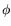about the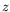-axis: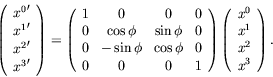(5.140)

The matrix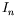has all zero elements accept for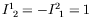, or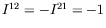. Thus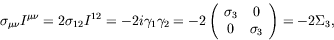(5.141)

Therefore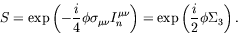(5.142)

For a rotation about an arbitrary axis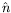, we write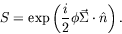(5.143)

The appearance of the half-angle is an expression of the double-valuedness of the spinor law of rotation; it takes a rotation of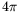to return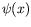to its original value. This is a characteristic of 1/2 integer spin. Therefore physical observables in the Dirac theory must be bilinear, or an even power in. By using the rotation operator upon the solution for the Dirac particle at rest and polarized in the-direction, it is possible to form states of any arbitrary direction. Since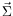is hermitian,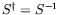for spatial rotations. We shall henceforth call the wave function of the Dirac theory a spinor.Next: Lorentz Boosts Up: Proof of Covariance Previous: Proof of Covariance
Douglas M. Gingrich (gingrich@ ualberta.ca)
2004-03-18